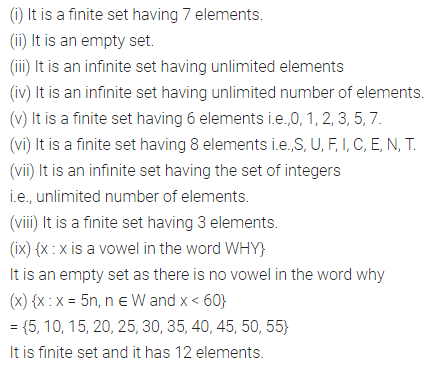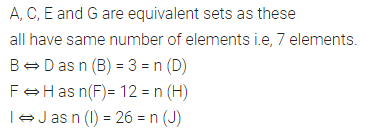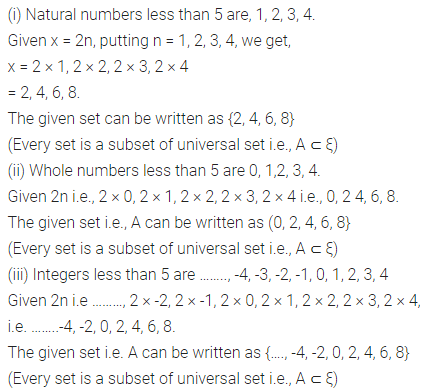# ML Aggarwal Class 7 Solutions for ICSE Maths Chapter 5 Sets Ex 5.2

## ML Aggarwal Class 7 Solutions for ICSE Maths Chapter 5 Sets Ex 5.2

Question 1.
Classify the following sets into empty set, finite set and infinite set. In case of (non-empty) finite sets, mention the cardinal number.
(i) {all colours of a rainbow}
(ii) {x | x is a prime number between 7 and 11}
(iii) {multiples of 5}
(iv) {all straight lines drawn in a plane}
(v) {x | x is a digit in the numeral 550131527}
(vi) {x | x is a letter in word SUFFICIENT}
(vii) {x | x = 4n, n ∈ I and x < 10}
(viii) {x | x ∈ N, x is a prime factor of 180}
(ix) {x : x is a vowel in the word WHY}
(x) {x : x = 5n, n ∈ W and x < 60}
Solution:Question 2.
Which of the following describe the same sets:
(i) {vowels of English alphabet} and {e, a, u, i, o}
(ii) {a, b, d} and {d, a, b, b}
(iii) {letters of PUPPET} and {E, T, P, U}
(iv) {1, 2, 3} and {2, 3, 4}
(v) {1, 2, 3, 4, 5} and {x | x ∈ N, x ≤ 5}
Solution:Question 3.
Find pairs/groups of equal sets from the following sets:
A = {0, 1, 2, 3}
B = {x : x2 < 10, x ∈ W}
C = {letters of word FOLLOW}
D = {days of a week}
E = {x | x ∈ W, x < 4}
F = {letters of word FLOW}
G = {Monday, Tuesday, ……… , Sunday}
H = {letters of word WOLF}
Solution:Question 4.
Find pairs/groups of equivalent sets from the following sets.
A = {colours of a rainbow}
B = {letters of word GOOD}
C = {x : x is a digit in the numeral 371011489}
D = {letters of word TOM}
E = {x : x ∈ I, x2 < 10}
F = {months of a year}
G = {days of a week}
H = {x | x = 3n, n ∈ W and n < 12}
I = {all even numbers between 1 and 53}
J = {all letters of English alphabets}
Solution:Question 5.
In the following, find whether A ⊂ B or B ⊂ A or none of these:
(i) A = { 1, 2, 3}, B = {2, 3, 3, 3, 1, 3}
(ii) A = {2, 4, 6,….}, B = {all natural numbers}
(iii) A = {x | x ∈ I, x2 < 20}, B = {0, 1, 2, 3, 4}
(iv) A = {letters of KING},
B = {letters of QUEEN}
Solution:Question 6.
State whether each of the following statement is true or false for the sets A and B where
A = {letters of CLOUD} and B = {letters of KOLKATA}
(i) A ⊂ B
(ii) B ⊂ A
(iii) A ↔ B
Solution:Question 7.
Write all the subsets of the following sets:
(i) Φ
(ii) {3, 5}
(iii) {2, 4, 6}
Solution:Question 8.
If A = {x : x = 2n, n < 5}, then find A when
(i) ξ = N
(ii) ξ = W
(iii) ξ = I
Solution: